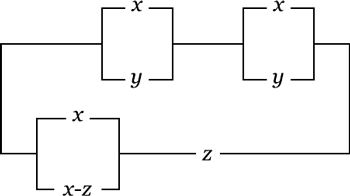MORE IN Engineering Mathematics - I
RGPV First Year Engineering (Set B) (Semester 2)
Engineering Mathematics - I
May 2014
Total marks: --
Total time: --
INSTRUCTIONS
(1) Assume appropriate data and state your reasons
(2) Marks are given to the right of every question
(3) Draw neat diagrams wherever necessary

1 (a) Expand $\log \dfrac {1+x}{1-x}$ in powers of x using Maclaurin's theorem.
2 M
1 (b) Define homogeneous functions and composite function and establish the Euler's theorem on homogeneous function.
2 M
1 (c) Find the extreme values of the function x3 + y2 - 3 axy.
3 M
Answer any one question from Q1. (d) & Q1. (e)
1 (d) If the sides and angles of a triangle ABC vary in such a way that its circum radius remains constant, prove that $\dfrac{da}{\cos A}+ \dfrac {db}{\cos B}+ \dfrac {dc}{\cos C}=0$
7 M
1 (e) Prove that the radius of curvature for the catenary Y=c cosh (x/c) is equal to the portion of the normal intercepted between the curve and the x-axis and that it varies as the square of the ordinate.
7 M

2 (a) Define Gamma function and Beta function and also establish the symmetry of Beta function.
2 M
2 (b) Evaluate the following integral by changing the order of integration: $\int^{1}_{0}\int^{c}_{c'}\dfrac {dydx}{\log y}$
2 M
2 (c) Evaluate by definition of definite integral as the limit of a sum $\int^{b}_{a}\sin x \ dx$
3 M
Answer any one question from Q2. (d) & Q2. (e)
2 (d) Find the volume bounded by the cylinder x2 + y2 = 4 and the plans y + z = 4 and z=0.
7 M
2 (e) Prove that: $\lim_{n\rightarrow \infty} \left [\left(1+ \dfrac{1^2}{n^2 }\right) \left(1+ \dfrac{2^2}{n^2}\right)\left(1+\dfrac{3^2}{n^2}\right)...\left(1+\dfrac{n^2}{n^2}\right)\right ]^{\frac{1}{4}}\\[2ex]=2e^{\frac {x-4}{2}}$
7 M

3 (a) Define the order and degree of a differential equation with one example also explain that the elimination of n arbitary constants from an equation leads us to which order derivative and hence a differential equation of which order.
2 M
3 (b) $Solve \ -ydx+xdy= \sqrt{x^2+y^2}dx$
2 M
3 (c) A bacteria population is known to have a rate of growth to itself. If between noon and 2 pm the population triples, at what time, no controls being exerted should becomes 100 times what it was at soon.
3 M
Answer any one question from Q3. (d) & Q3. (e)
3 (d) $Solve \ x^3\dfrac {d^3y}{dx^3}+3x^2\dfrac {d^2y}{dx^2}+x\dfrac {dy}{dx}+y=x+\log x.$
7 M
3 (e) Solve the following differential equation by using the method of variation of parameters. $\dfrac {d^2y}{dx^2}-2\dfrac {dy}{dx}+2y=e^x \tan x$
7 M

4 (e) Find the eigen values of A and using Cayley-Hamilton theorem. Find An (n is a positive integer); given that $\begin{bmatrix}1&2 \\ 4&3 \end{bmatrix}$
7 M
4 (a) Determine the rank of the following matrix $\begin{bmatrix}4 &2 &3 \\ 8&4 & 6\\ -2&-1 &-1.5 \end{bmatrix}$
2 M
4 (b) Solve the system of equation using matrix method. X+3y-2z=0
2x-y+4z=0
x-11y+14z=0
2 M
4 (c) If A is a non-singular matrix, prove that the eigen values of A-1 are the reciprocal of the eigen values of A.
3 M
Answer any one question from Q4. (d) & Q4. (e)
4 (d) Find the eigen values eigen vectors of the matrix $\begin{bmatrix}-2&2 &-3 \\ 2&1 &- 6\\ -1&-2 &0\end{bmatrix}$
7 M

5 (a) What do you mean by logical equivalence and prove that the statement (p?q) ? (?p ??q) is a contradiction.
2 M
5 (b) For a simple graph of n vertices, the number of edge is $\dfrac {1}{2} n (n-1)$
2 M
5 (c) Simplify the following circuit3 M
Answer any one question from Q5. (d) & Q5. (e)
5 (d) A simple graph with n vertices and k compoents can have at most $\dfrac {(n-k)(n-k+1)}{2}$ edges.
7 M
5 (e) Express the following functions into disjunctive normal form f(x,y,z)=x.y'+x.z+x.y
7 M

More question papers from Engineering Mathematics - I